## TN State Board 11th Chemistry Important Questions Chapter 4 Hydrogen

Question 1.
Mention the properties in which ortho and para hydrogen differ.
(i) The boiling point and melting point para hydrogen is lower than that of ortho hydrogen.
(ii) Para hydrogen has zero magnetic moment while ortho hydrogen has magnetic moment twice as that of proton.

Question 2.
Explain the laboratory preparation of hydrogen.
Hydrogen is conveniently prepared in laboratory by the reaction of metals, such as zinc, iron, tin with dilute acid.

Zn + 2 HCl → ZnCl2 + H2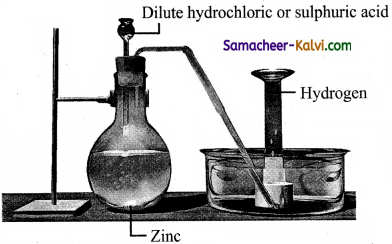Question 3.
Explain steam -reforming of hydrocarbons to produce hydrogen gas.
In this method hydrocarbon such as methane is mixed with steam and passed over nickel catalyst in the range 800 – 900°C and 35 atm pressures.
CH4 + H2O → CO + 3H2

Question 4.
What is water gas? How is it produced?
Water gas is a mixture of carbon monoxide (CO) and hydrogen (H2), Steam is passed over a red-hot coke to produce carbon monoxide and hydrogen.

Question 5.
How will you convert carbon monoxide in water gas to carbondioxide?
The carbon monoxide of the water gas can be converted to carbon dioxide by mixing the gas mixture with more steam at 400°C and passed over a shift converter containing iron/copper Catalyst. This reaction is called as water-gas shift reaction.
CO + H2O → CO2 + H2
The CO2 formed in the above process is absorbed in a solution of potassium carbonate.
CO2 + K2CO3 + H2O → 2 KHCO3Question 6.
How is heavy hydrogen D2 produced?
Prolonged electrolysis of heavy water produces heavy hydrogen. The electrolysises continued until the resulting solution becomes enriched in heavy water. Further electrolysis of the heavy water gives deuterium.
2D2O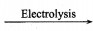2D2 + O2

Question 7.
How is Tritium prepared?
It is artificially prepared by bombarding lithium with slow neutrons in a nuclear fission reactor. The nuclear transmutation reaction for’ this process is as follows.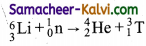Question 8.
Give the reaction which takes place in H2 – O2 fuel cells.
2H2 + O2 → 2H2O. This reaction is explosive and releases a lot of energy, which is used in fuel cells to generate electricity.Question 9.
What is the order of reactivity of halogens towards hydrogen?
The order of reactivity is F2 > Cl2 > Br2 > I2.

H2 + F2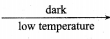2HF
H2 + Cl2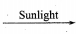2HCl
H2 + Br2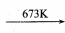2HBr
H2 + I2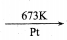2HI

Question 10.
Mention the oxidation state of hydrogen in hydrides. Give three examples of reactive metal hydrides.
The oxidation state of hydrogen in hydrides is -1.
The reactive metal hydrides as LiH, NaH, CaH.

Question 11.
Name two hydrides which are used as reducing agents in organic chemistry. How are they prepared?
Lithium aluminium hydride (Li AlH4) and sodium borohydride (NaBH4) are used as a reducing agent in organic chemistry. They are prepared as follows:
4 LiH + AlCl → Li [AlH4] + 3 LiCl
4 NaH + B(OCH3)3 → Na [BH4] + 3 CH3ONaQuestion 12.
Give example of a reaction where H2 acts as a reducing agent.
Hydrogen itself acts as a reducing agent. In the presence of finely divided nickel, it adds to the unsaturated organic compounds to form saturated compounds.
HC ≡ CH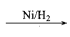H2C = CH2H3C – CH3

Question 13.
How does D2 react with O2 and halogen? Write balanced equation.
They form heavy water and halides.
2D2 + O2 → 2D2O
D2 + X2 → 2DX [X = F, Cl, B and I]

Question 14.
Give a brief account of exchange reaction of D2O.
In these reactions, hydrogen in the reactants is replaced by D.
2NaOH + D2O → 2NaOD + HOD
HCl + D2O → DCl + HOD
NH4Cl + 4D2O → ND4Cl + 4HOD

Question 15.
Mention the use of H2 in metallurgy.
In metallurgy, hydrogen can be used to j reduce many metal oxides to metals at high 1 temperatures.
CuO + H2 → Cu + H2O
WO3 + 3H2 → W + 3H2OQuestion 16.
Mention the industrial importance of hydrogen.
(i) Ammonia is synthesized in a large scale by Haber process. The reaction is
N2 + 3H2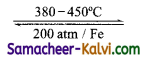2NH3
(ii) Ammonia is used in the manufacture of chemicals such as nitric acid, fertilises and explosives.
(iii) It can be used to manufacture the industrial I solvent, methanol from carbon monoxide using copper as catalyst.
CO + 2H2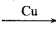CH3OH

Question 17.
Give a brief account of the action of water on metals.
(i) The most reactive metals i.e., the alkali metals, decompose water even in cold with the evolution of hydrogen leaving an alkali metal hydroxide solution.
2Na + 2 H2O → 2 NaOH + H2

(ii) The group 2 metals (except beryllium) react in a similar way but less violently, The hydroxides are less soluble in water than those of Group 1.
Ba + 2H2O → Ba(OH)2 + H2

(iii) Some transition metals react with hot water or steam to form the corresponding oxides. For example, steam passed over red hot iron results in the formation of iron oxide with the release of hydrogen.
3Fe + 4H2O → Fe3O4 + H2

(iv) Lead and copper decompose water only at a red hot. Silver, gold, mercury and platinum do not have any effect on water.Question 18.
Give a brief account of the action of water on non-metals.
(i) Fluorine decomposes cold water.
2F2 + 2H2O2 → 2H2F2 + O2
3F2 + 3H2O2 → 2H2F2 + O3
(ozonised oxygen)

(ii) Chlorine decomposes cold water forming j HC1 and HOCl (hypochlorous acid) j
Cl2 + H2O → 2HCl + HOCl.

Question 19.
What are the causes for the hardness of water?
The presence of Ca+2 and Mg+2 ions and that of metal salt in the form of bicarbonate, chloride and sulphate ions makes water hard.

Question 20.
How is temporary hardness removed?
This can be removed by boiling the hard water followed by filtration. Upon boiling, these salts decompose into insoluble carbonate which leads to their precipitation. The magnesium carbonate thus formed further hydrolysed to give insoluble magnesium hydroxide.
Ca(HCO3)2 → CaCO3 + H2O + CO2
Mg(HCO3)2 → MgCO3 + H2O+ CO2
MgCO3 + H2O → Mg(OH)2 + CO2
The resulting precipitates can be removed by filtration.Question 21.
Explain Clark’s method of remaining temporary hardness of water.
The Clark’s method uses, calculated amount of lime to hard water containing the magnesium and calcium and the resulting carbonates and hydroxides are filtered-off.
Ca(HCO3)2 + Ca(OH)2 → 2CaCO3 + 2H2O
Mg (HCO3)2 + 2Ca(OH)2 → 2CaCO3 + Mg(OH)2 + 2 H2O

Question 22.
How is permanent hardness in hardwater is removed?
Permanent hardness of water is due to the presence of soluble salts of magnesium and calcium in the form of chlorides and sulphates in it. It can be removed by adding washing soda, which reacts with these metal (M = Ca or Mg) chlorides and sulphates in hard water to form insoluble carbonates.
MCl2 + Na2CO3 → MCO3 + 2 NaCl
MSO4 + Na2CO3 → MCO3 + Na2SO4

Question 23.
Give the chemical properties of heavy water and water.
Heavy water is chemically similar to ordinary water (H2O). However D2O reacts more slowly than H2O in chemical reactions.
(i) When compounds containing hydrogen are treated with D2O, hydrogen undergoes an exchange for deuterium,
2NaOH + D2O → 2NaOD + HOD .
HCl + D2O → DCl + HOD
NH4Cl + 4D2O → ND4Cl + 4HOD

These exchange reactions are useful in determining the number of ionic hydrogens present in a given compound. For example, when D2O is treated with of hypo-phosphorus acid only one hydrogen atom is exchanged with deuterium. It indicates that, it is a monobasic acid.

H3PO2 + D2O → H2DPO2 + HDO

It is also used to prepare some deuterium compounds:

Al4C3 + 12 D2O → 4 Al(OD)3 3CD4
CaC2 + 2 D2O → Ca(OD)2 + C2D2
Mg3`N2 + 6 D2O → 3 Mg(OD)2 + 2 ND3
Ca3P2 + 6 D2O → 3 Ca(OD)2 + 2 PD3Question 24.
What do you understand by 100 volume H2O2?
This means 100 ml of oxygen is liberated by 1 ml of H2O2 solution as heating at STP.

Question 25.
An ice cube atO°C is placed in some liquid water at 0°C, the ice cube sinks – Why?
The density of ice is lower than that of water. Hence it sinks in liquid water.

Question 26.
Calculate (i) Concentration of H2O2 in g/L,
(ii) Normally and
(iii) Molarity of a 10 vol solution of H2O2.
(i)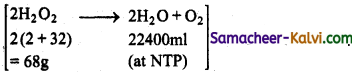22400 ml of oxygen is obtained at NTP from = 68g of H2O2
10 ml of oxygen is obtained at NTP from = $$\frac{68}{22400}$$ = 0.03035 g of H2O2
1 m/ of H2O2 contains = 0.03035 g H2O2
100 ml of H2O2 contains = 0.03035 × 100 = 3.035 g of H2O2
or
the solution is 3.035%
or
concentration of ‘10 vol’ solution = 3.035 × 10 = 30.35 g/litre.

(ii) Normality (N) = $$\frac{\text { Concentration in } \mathrm{g} / \mathrm{L}}{\text { Equivalent mass }}$$
Equivalent mass of H2O2 = $$\frac{\text { Molecular mass }}{2}=\frac{34}{12}$$ = 17
Normality (N) = $$\frac{30.35}{17}$$ = 1.785
Normality of 10 volume solution = 1.785 N
[Relationship between volume strength and normality]
Let volume strength of H2O2 solution be ‘V’.
i.e., ‘ V’ litre of O2 is given by 1L of H2O2 at NTP.
g/L of H2O2 = $$\frac{68}{22.4}$$ × V
N = $$\frac{\text { strength }}{\text { Equivalent mass }}$$
= $$\frac{68}{22.4} \times \mathrm{V} \times \frac{1}{17}$$

‘V’ = 5.6 × Normality
Similarly ‘V’ = 11.2 × molarityQuestion 27.
Give a brief account of ionic hydrides.
These are hydrides composed of an electropositive metal, generally, an alkali or alkaline-earth metal, except beryllium and magnesium, formed by transfer of electrons from metal to hydrogen atoms. They can be prepared by the reaction of elements at about 400° C. These are salt-like, high-melting, white crystalline solids having hydride ions (H) and metal cations Mn+ where ‘n’ is the valency of the metal.
2 Li + H2 → 2 LiH

Question 28.
Give a brief account of covalent hydrides.
Covalent hydrides are compounds in which hydrogen is attached to another element by sharing of electrons. Covalent hydrides are further divided into three categories, viz., electron precise (CH4, C2H6, SiH4, GeH4), electron-deficient (B2H6) and electron- rich hydrides (NH3, H2O). Since most of the covalent hydrides consist of discrete, small molecules that have relatively weak intermolecular forces, they are generally gases or volatile liquids.

Question 29.
Give a brief account of metallic (interstitial) hydrides.
In metallic hydrides hydrogen occupies the interstitial sites (voids) of their crystal lattice. Hence, they are called interstitial hydrides; these hydrides show properties similar to parent metals and hence they are also known as metallic hydrides. Most of the hydrides are non-stoichiometric with variable composition (TiH1.5 – 1.8 and PdH0.6 – 0.8). Some are relatively light, inexpensive and thermally unstable which make them useful for hydrogen storage applications. Electropositive metals and some other metals form hydrides with the stoichiometry MH or sometimes MH2 (M = Ti, Zr, Hf, V, Zn).Question 30.
Explain how hydrogen bond influence the properties of compound which can form hydrogen bond.
Hydrogen bond is weaker than covalent bond but stronger than vanderwaals attraction compounds in which hydrogen bonding occur will have higher boiling point, melting point and solubility.
eg: H2O has a higher boiling point than H2S. CH3OH is soluble in water because it can form hydrogen bonding with water.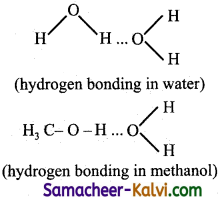Question 31.
Explain (i) intermolecular hydrogen bond and
(ii) intramolecular hydrogen bond.
(i) Intermolecular hydrogen bonds occur between two separate molecules. They can occur between any numbers of like or unlike molecules as long as hydrogen donors and acceptors are present in positions which enable the hydrogen bonding interactions. For example, intermolecular hydrogen bonds can occur between ammonia molecule themselves or between water molecules themselves or between ammonia and water.

(ii) Intramolecular hydrogen bonds are those which occur within a single molecule.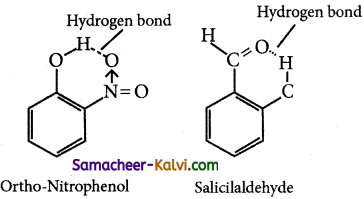Question 32.
Explain the structure of ice.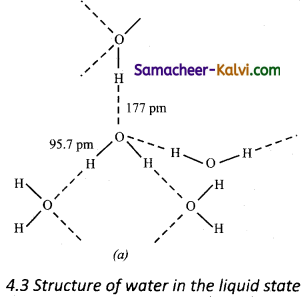In ice, each atom is surrounded tetrahedrally by four water molecules through hydrogen bonds. That is, the presence of two hydrogen atoms and two lone pairs of electron on oxygen atoms in each water molecule allows formation of a three-dimensional structure. This arrangement creates an open structure having a number of vacant spaces. As a result the density of ice is less than that of liquid.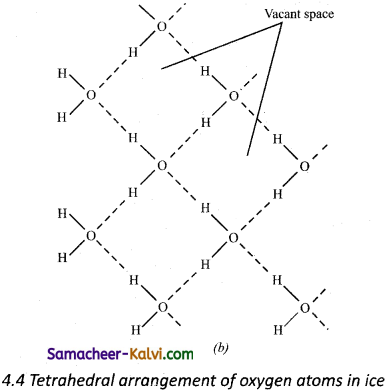Tetrahedral arrangement of oxygen atoms in ice The above property of water is very helpful in aquatic life. In extreme cold conditions, the upper layer of sea or lake freezes. The ice layer being lighter does not sink and thereby sea animals or lake animals can live safely in water under these conditions.Choose the correct answer:

Question 1.
The element which does not contain a neutron is:
(a) hydrogen
(b) helium
(c) chlorine
(d) none of the above
(a) hydrogen

Question 2.
The number of electrons, protons and neutrons in protium, deuterium and tritium are respectively:
(a) 1, 1, 0 ; 1, 1, 1 ; 1, 1, 2
(b) 1, 1, 1 ; 1, 1, 0; 1, 1, 2
(c) 1, 1, 2; 1, 1, 0; 1, 1, 1
(d) 1, 1, 1; 1, 1, 0; 1, 1, 2
(a) 1, 1, 0 ; 1, 1, 1 ; 1, 1, 2

Question 3.
Among the isotopes of hydrogen, which has higher mass and radio active?
(a) Protium
(b) Tritium
(c) Deuterium
(d) both deuterium and tritium
(b) TritiumQuestion 4.
Choose the incorrect statement:
(a) The boiling points of both deuterium and tritium are higher than that of protium.
(b) The inter nuclear distances between the two bonded atoms are different in all the isotopes.
(c) Enthalpy of dissociation is high in tritium
(d) both (b) and (c)
(b) The inter nuclear distances between the two bonded atoms are different in all the isotopes.

Question 5.
Normal hydrogen consists of:
(a) 75% ortho form and 25% para form
(b) 25% ortho form and 75% para form
(c) 80% ortho form and 20% para form
(d) 20% ortho form and 80% para form
(a) 75% ortho form and 25% para form

Question 6.
Choose the correct statement with regard to ortho and para hydrogen.

1. Both have similar chemical properties but differ in some physical properties.
2. The boiling points of normal, ortho and para hydrogens are the same.
3. The nuclear spin in both forms are the same.
4. Para hydrogen has zero magnetic moment.

(a) 1, 2
(b) 1, 4
(c) 2, 3
(d) 2, 4
(b) 1, 4Question 7.
CO2 + K2CO3 + H2O → X. The product X is:
(a) KHCO3
(b) CO2
(c) H2CO3
(d) KOH
(b) CO2
Hint:
CO2 is absorbed by a solution of K2CO3.

Question 8.
Which of the following dissociate more readily?
(a) H2O
(b) D2O
(c) T2O
(d) H2 all dissociate to the same extent
(a) H2O
Hint:
Because of smaller size of hydrogen atom compared to D. i.e., H – O bond is weaker than D – O bond.

Question 9.
Hydrogen resembles halogens in many respects for which several factors are responsible of the following factors which one is most important in this respect.
(a) Its tendency to lose an electron and form a cation.
(b) Its tendency to gain an electron in its valence shell to attain stable electronic configuration.
(c) Its low electron gain enthalpy.
(d) Its small size.
(b) Its tendency to gain an electron in its valence shell to attain stable electronic configuration.Question 10.
Metal hydrides are ionic, covalent or molecular in nature. Among LiH, NaH, KH, RbH, CsH the correct order of increasing ionic character is:
(a) LiH > NaH > CsH > KH > RbH
(b) LiH < NaH< KH < RbH < CsH
(c) RbH > CsH > NaH > KH > LiH
(d) NaH > CsH > RbH > LiH > KH
(b) LiH < NaH< KH < RbH < CsH
Hint:
Ionic character increases as the size of the atoms increases or the electronegativity of the atom decreases.

Question 11.
Which of the following is electron precise hydride?
(a) B2H6
(b) NH3
(c) H2O
(d) CH4
(d) CH4
Hint:
Electron precise hydrides contain, exact number of electrons to form normal covalent bond.

Question 12.
Consider the reactions:
(i) H2O2 + 2HI → I2 + 2H2O
(ii) HOCl + H2O2 → H3O+ + Cl + O2
Which of the following statements is correct about H2O2 with reference to the above reactions?
(a) an oxidising agent in both (i) and (ii)
(b) an oxidising agent in (i) and reducing agent in (ii)
(c) a reducing agent in (i) and oxidising agent in (ii)
(d) a reducing agent in both (i) and (ii)
(b) an oxidising agent in (i) and reducing agent in (ii)
Hint:
The thumb-rule for oxidising behaviour of H2O2 is:
H2O2 acts as an reducing agent, if O2 is evolved and H2O2 acts as a oxidising agent O2 is NOT evolved.Question 13.
Which of the following reactions increases the production of di-hydrogen from synthesis gas?
(a) CH4 (g) + H2O(g)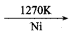CO + 3H2 (g)
(b) C (s) + R2O (g)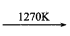CO (g) + H2 (g)
(c) CO (g) + H2O (g)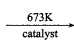CO (s) + H2 (g)
(d) C2H6 (g) + 2HO (g)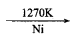2CO(g) + 5H2 (g)
(c) CO (g) + H2O (g)CO (s) + H2 (g)

Question 14.
When sodium peroxide is treated with dilute sulphuric acid, the products are:
(a) sodium sulphate and water
(b) sodium sulphate and oxygen
(c) sodium sulphate, hydrogen and oxygen
(d) sodium sulphate and hydrogen peroxide
(d) sodium sulphate and hydrogen peroxide
Hint:
Na2O2 + H2SO4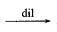Na2SO4 + H2O2

Question 15.
Which of the following compounds is a peroxide?
(a) KO2
(b) BaO2
(c) MnO2
(d) NO2
(b) BaO2
Hint:
Peroxides produce H2O2 an treatment with dilute acid. The rest of the compound does not produce H2O2 with acid.Question 16.
Ag2O+ H2O2 → 2Ag + H2O + O2. In this reaction H2O2 acts as:
(a) reducing agent
(b) oxidising agent
(c) bleaching agent
(d) none of these
(a) reducing agent
Hint:
Refer thumbs rule in Q.No. 12

Question 17.
Bond angles in H—O—H and H—O—O—H in water and H2O2 respectively are:
(a) 104.5°, 104.5°
(b) 94.8°, 94.8°
(c) 104.5°, 94.8°
(d) 94.8°, 104.5°
(c) 104.5°, 94.8°

Question 18.
When zeolite, which is hydrated sodium aluminium silicate is treated with hard water, the sodium ions are exchanged with:,
(a) H+ ions
(b) Ca+2 ions
(c) SO4-2 ions
(d) M+2 ions
(a) H+ ionsQuestion 19.
The reagent used for softening of the temporary hardness of water is / are:
(i) Ca3(PO4)2
(ii) Ca(OH)2
(Hi) Na2CO3
(iv) NaOCl
(a) (ii) and (iii)
(b) (i) and (iii)
(c) (ii) and (iv)
(d) (i) and (iv)
(a) (ii) and (iii)
Hint:
Ca(OH)2 and Na2CO3 react with bicarbonates of Ca and Mg present in temporary hard water to form corresponding insoluble carbonates which an filtration give soft water.

Question 20.
In which of the following reactions H2O2 act as a

(i) H2O2 + 2H+ + 2e → 2H2O
(ii) H2O2 – 2e → O2 + 2H+
(iii) H2O2 + 2e → 2OH
(iv) H2O2 + 2OH – 2e → O2 + 2H2O

(a) (ii) and (iv)
(b) (i) and (ii)
(c) (iii) and (iv)
(d) (i) and (iii)
(b) (i) and (ii)

Question 21.
Match the entities of column I with appropriate entities of column II and choose the correct option out of the following options (a),(b),(c) and (d) given at the end of the questions.

 Column I Column II (i) BeH2 (A) Complex (ii) AsH3 (B) Interstitial (iii) LaH3 (C) Covalent (iv) LiAlH4 (D) Polymeric

(a) (i) – (D), (ii) – (C), (iii) – (A), (iv) – (B)
(b) (i) – (A), (ii) – (D), (iii) – (B), (iv) – (C)
(c) (i) – (C), (ii) – (B), (iii) – (D), (iv) – (A)
(d) (i) – (D), (ii) – (C), (iii) – (B), (iv) – (A)
(d) (i) – (D), (ii) – (C), (iii) – (B), (iv) – (A)Question 22.
Match the following:

 Column I Column II (i) Electrolysis of water produces (A) Atomic reactor (ii) Lithium aluminium hydride is used as (B) Polar molecule (iii) Hydrogen chloride acts as (C) Reducing agent (iv) Heavy water is used in (D) Hydrogen and oxygen

(a) (i) – (D), (ii) – (C), (iii) – (B), (iv) – (A)
(b) (i) – (C), (ii) – (B), (iii) – (A), (iv) – (D)
(c) (i) – (C), (ii) – (A), (iii) – (D), (iv) – (B)
(d) (i) – (B), (ii) – (D), (iii) – (C), (iv) – (A)
(d) (i) – (B), (ii) – (D), (iii) – (C), (iv) – (A)

Question 23.
Select the odd man out from the following: an amphoteric oxide is:
(a) Na2O
(b) BeO
(c) Cl2O7
(d) MgO
(b) BeO

Question 24.
Select the odd man out from the following: An electron precise hydride is:
(a) B2H6
(b) NH3
(c) H2O
(d) CH4
(d) CH4Question 25.
Among the given hydrides, identify the pair in which the first one is ionic and the second is metallic hydride,
(a) LiH7, BeH2
(b) BeH2, LaH2.87
(c) NaH , LaH2.87
(d) CH4, BeH2
(c) NaH , LaH2.87

Question 26.
Which of the following pairs of substances on reaction will not evolve H2 gas?
(a) Fe and H2SO4 (aq)
(b) Cu and HCl (aq)
(c) Na and alcohol
(d) Fe and steam
(b) Cu and HCl (aq)

Question 27.
Assertion :
The water gas shift reaction can be used to increase the amount of syngas mixture.
Reason :
In this reaction, carbon monoxide reduces steam to hydrogen.
(a) If both assertion and reason are true and reason is the correct explanation of the assertion. .
(b) If both assertion and reason are true but reason is not the correct explanation of the assertion.
(c) If assertion is true but reason is false.
(d If both assertion and reason are false.
(a) If both assertion and reason are true and reason is the correct explanation of the assertion.Question 28.
Assertion :
Chlorine reacts more rapidly with H2 than D2.
Reason :
D – Cl bond is stronger than H – Cl bond.
(a) If both assertion and reason are true and reason is the correct explanation of the assertion.
(b) If both assertion and reason are true but reason is not the correct explanation of the assertion.
(c) If assertion is true but reason is false.
(d) If both assertion and reason are false.
(b) If both assertion and reason are true but reason is not the correct explanation of the assertion.

Question 29.
Assertion :
Electrolysis of molten CaH2 produces hydrogen gas at anode.
Reason :
In CaH2, hydrogen is present in the form of H ion.
(a) If both assertion and reason are true and reason is the correct explanation of the assertion.
(b) If both assertion and reason are true but reason is not the correct explanation of the assertion.
(c) If assertion is true but reason is false.
(d) If both assertion and reason are false.
(a) If both assertion and reason are true and reason is the correct explanation of the assertion.

Question 30.
Assertion :
H2O is the only hydride of group 16 which is a liquid at room temperature.
Reason :
In ice, each hydrogen atom is surrounded by two covalent bonds and two hydrogen bonds.
(a) If both assertion and reason are true and reason is the correct explanation of the assertion.
(b) If both assertion and reason are true but reason is not the correct explanation of the assertion.
(c) If assertion is true but reason is false.
(d) If both assertion and reason are false.
(b) If both assertion and reason are true but reason is not the correct explanation of the assertion.Question 31.
Assertion :
The O – O bond in H2O2 is shorter in H2O2 than that of O2F2.
Reason :
H2O2 is an ionic compound.
(a) If both assertion and reason are true and reason is the correct explanation of the assertion.
(b) If both assertion and reason are true but – rea§pn is not the correct explanation of the assertion.
(c) If assertion is true but reason is false.
(d) If both assertion and reason are false.
(d) If both assertion and reason are false.

Question 32.
Choose the incorrect statement from the following:
(a) HF is an electron rich hydride.
(b) Water softened by Permutit process is not demineralised water since it still contains sodium salts.
(c) H2O2 does not decompose NaHCO3 since it is weaker acid than H2CO3.
(d) In H2O2, the two O – H bonds lie in the same plane.
(d) In H2O2, the two O – H bonds lie in the same plane.Question 33.
Consider the reactions:

(I) H2O2 + O3 → H2O + 2O2
(II) H2O2 + Ag2O → 2Ag + H2O + O2

The role of H2O2 in the above reaction is respectively:
Choose the correct statement from the following:
(a) Oxidising in (I) and reducing in (II)
(b) Reducing in (I) ancl oxidising in (I)
(c) Reducing in (I) and (II)
(d) Oxidising in (I) and (II)
(c) Reducing in (I) and (II)

Question 34.
What is incorrect about H2O2?
(a) Acts as both oxidising and reducing agent.
(b) Two O – H bonds lie in the same plane.
(c) Pale and blue liquid.
(d) Can be oxidised by O3.
(b) Two O – H bonds lie in the same plane.Question 35.
Which of the following statements is incorrect with respect to hydrogen?
(a) Hydrogen has three isotopes of which protium is the most common.
(b) In hydrides, hydrogen exists as an anion.
(c) Di hydrogen does not act as a reducing agent.
(d) Hydrogen ion exists as H3O+ Ions in aqueous solution.
(c) Di hydrogen does not act as a reducing agent.

Question 36.
Which of the following statements are correct?

(i) The hydrogen bonding exists in hydrogen fluoride is intra molecular hydrogen bond.
(ii) In alkaline solutions, H2O2 acts as a reducing agent and it gets reduced to H2O and O2.
(iii) H2O2 is an acid.
(iv) H2O2 is also bleaching agent.

(a) (ii), (iii), (iv)
(b) (ii)and (iii)
(c) (i) and (iii)
(d) All